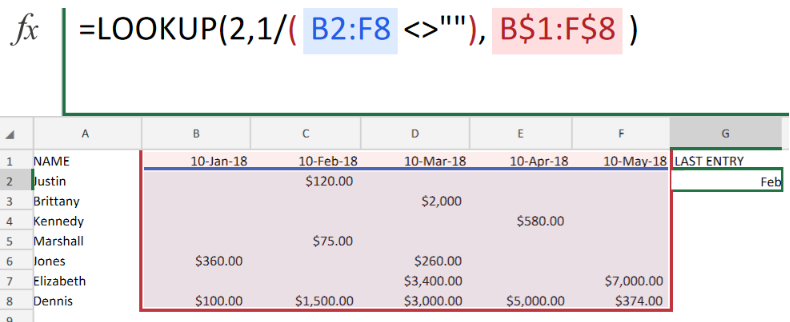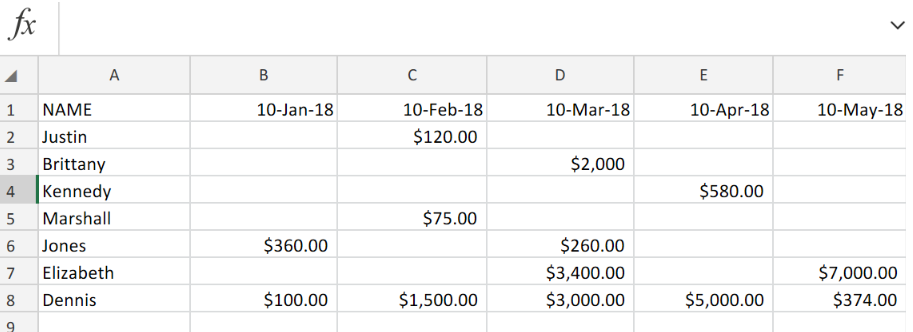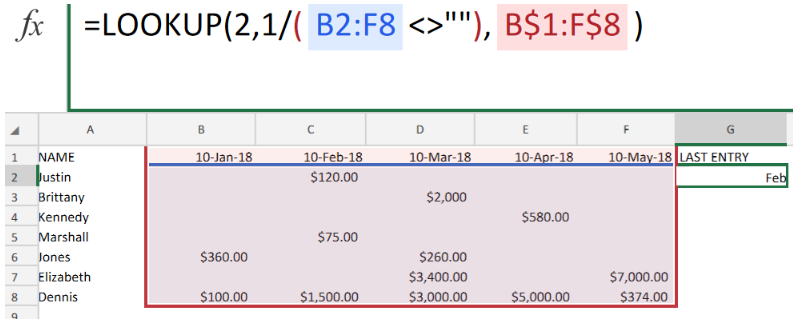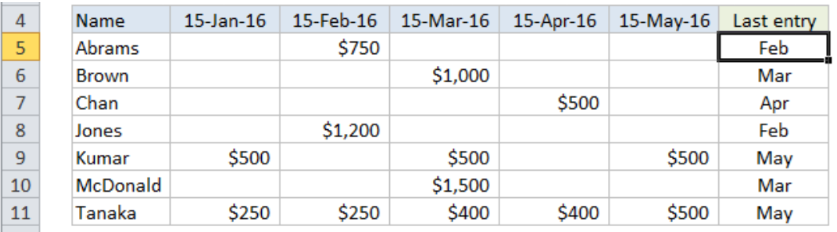Get instant live expert help with Excel or Google Sheets“My Excelchat expert helped me in less than 20 minutes, saving me what would have been 5 hours of work!”

#### Post your problem and you’ll get expert help in seconds.

Your message must be at least 40 characters
Our professional experts are available now. Your privacy is guaranteed.

# Get Date Associated with Last EntryFigure 1. of Date Associated with Last Entry in Excel.

In order to determine a calendar date corresponding with the last data entry in our Excel sheet, we can used the Excel LOOKUP Function. This article will step through the process.

## Generic Formula

`=LOOKUP(2,1/(row<>""),header)`

Where row is the total range of cells containing the data entries and header is the range of cells containing the headers for the columns used in our worksheet.

## How to use the Excel LOOKUP Function.

We are now going to demonstrate the usage of the Excel LOOKUP Function in the following three simple steps;

1. Collect and arrange the data values in our Excel sheet. In the example illustrated below, we are working with sales entries along with corresponding calendar dates.Figure 2. of Sales Records with Calendar Dates.

1. For us to obtain the date associated with the last entry in our worksheet, we will enter the following formula into the formula bar for cell G2 in the example illustrated below.

`=LOOKUP(2,1/(B2:F8<>""),B\$1:F\$8)`Figure 3. of LOOKUP Function in Excel.

The formula will lookup the specified range of cells containing the calendar entries and automatically return the calendar date associated with the last entry.

1. Modify and copy the formula above into the other cells in the LAST ENTRY column of our worksheet, to get the desired results.Figure 4. of Date Associated with Last Entry in Excel.

## Note

• Our LOOKUP value = 2, and the largest value in the LOOKUP range is 1. Hence the LOOKUP Function will match the last 1in our specific cell range.

## Instant Connection to an Expert through our Excelchat Service

Our live Excelchat Service is here for you. We have Excel Experts available 24/7 to answer any Excel questions you may have. Guaranteed connection within 30 seconds and a customized solution for you within 20 minutes.

### Did this post not answer your question? Get a solution from connecting with the expert.Another blog reader asked this question today on Excelchat:
Solution examplesI'm trying to figure out how to have a value found in column c placed into column d if the value in column a equals that found in column b. Does that make sense?
Solved by I. H. in 40 minsWhen i do a VLOOKUP, I cannot autofill the formula for the rest of the table. It copies the initial cell that the VLOOKUP was done.
Solved by G. A. in 20 minsI need a formula (I think VLOOKUP) that will enable me to: Use data from one column, compare with another and tell me the difference. Im a teacher and want to work out the amount of marks needed to the next grade boundary. I've created the rest, but this has me stumped!
Solved by F. A. in 28 minshow do I do the same formula for different cells, but i want a different cells value in each of the formulas. For example, if i was trying to find the percentage grade of each student in a class of 300.
Solved by I. W. in 14 minsI'm not sure as to why my formula isn't working. I will send two files... Workbook 1.xlsx.xls Workbook 2.xlsm The problem is in Column H of Workbook 1.
Solved by E. H. in 42 mins## Subscribe to Excelchat.coAnother blog reader asked this question today on Excelchat: